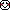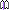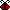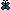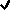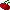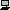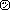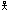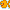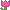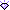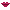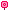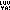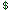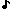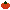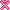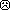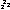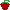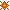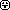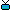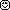kadyellebee.com is a site for all-things-kristine.

## Credits

my life is powered by Six Apart.

### Brain Teaser

As I started to clean some boxes out, I found the following brainteaser. I remember having such a fun time trying to solve it. If you can solve all 24 of them, I’ll put a special little linky to your site in my sidebar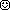(I’m gonna have to go reremind myself on some of these, too LOL!)

Brain Teaser Formulas
Example: 12 = E. in a D. (12 eggs in a dozen)

1. 26 = L. of the A.
2. 7 = D. of the W.
3. 1001 = A.N.
4. 12 = S. of the Z.
5. 54 = C. in a D. with the J.
6. 9 = P. in the S. S.
7. 88 = P. K.
8. 13 = S. on the A. F.
9. 32 = D.F and which W. F.
10. 18 = H. on a G. C.
11. 90 = D. in a R. A.
12. 200 = D. for P. G. in M.
13. 8 = S. on a S. S.
14. 3 = B. M. (S. H. T. R.)
15. 4 = Q. in a G.
16. 24 = H. in a D.
17. 1 = W. on a U.
18. 5 = D. in a Z. C.
19. 57 = H. V.
20. 11=P on a F. T.
21. 1000 = W. that a P. is W.
22. 29 = D. in F. in a L. Y.
23. 64 = S. on a C. B.
24. 40 = D. and N. of the G. F.

Have fun!!

I`ll do the ones I know now and e-mail them and the rest when I`ve worked them out